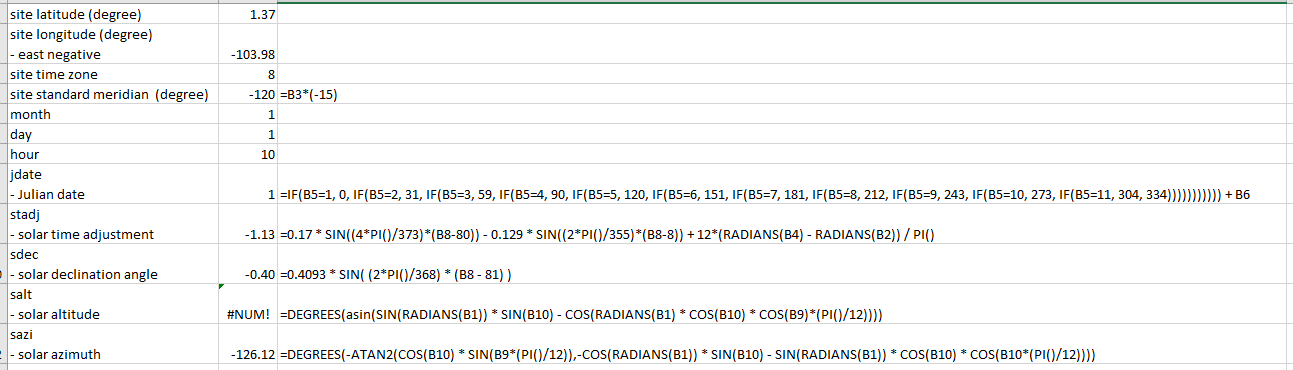Question-and-Answer Resource for the Building Energy Modeling Community
Get started with the Help page

sun altitude and azimuth calculation error following sun.c in Radiance source filesI'm trying to understand how Radiance calculate the position of sun at a particular hour.

Following the sun.c source code in the Radiance package at here, I calculated the values step by step, but got errors for solar altitude and azimuth:

The formula used in each step is stated beside the value:I have the following questions:

1. May I ask how solar altitude and azimuth are defined in Radiance?

2. the value feed into the asin() function for the calculation of solar altitude has an absolute value larger than 1. May I ask what went wrong in the Excel calculation if i didn't interpret the c file correctly?

3. Seems that only month and day are used for the calculation. May I ask why hour is not used in the calculation of solar altitude and azimuth?

The formula used are:

jdate = IF(B5=1, 0, IF(B5=2, 31, IF(B5=3, 59, IF(B5=4, 90, IF(B5=5, 120, IF(B5=6, 151, IF(B5=7, 181, IF(B5=8, 212, IF(B5=9, 243, IF(B5=10, 273, IF(B5=11, 304, 334))))))))))) + B6

sdec = 0.4093 * SIN( (2*PI()/368) * (B8 - 81) )

sazi = DEGREES(-ATAN2(COS(B10) * SIN(B9(PI()/12)),-COS(RADIANS(B1)) * SIN(B10) - SIN(RADIANS(B1)) * COS(B10) * COS(B10(PI()/12))))

edit retag close merge delete

@oat 1. Usually azimuth is calculated in degrees from true North. Solar altitude refers to the angle of the sun relative to the Earth's horizon also in degrees. I don't see any reason why it would be different in Radiance . 2. I think your problem is the site standard meridian relative to site longitude. The difference between them can't exceed +/- 7.5 degrees.(this is wrong assumption read below) 3. It might be that this function calculates only the solar noon. .(this is wrong assumption read below)

@Avi There's no reason that standard meridian and site longitude need to be within 7.5 degrees of each other. There are many places in the world where they do not, as time zone boarders do not run due north and south.

@Nathaniel Jones I see that. So the stadj is hours that had to be added to the local time to account for distance from meridian.

I've never looked at the Radiance code, so I can't tell what stadj does, but my guess is that it corrects for the difference between the solar time and the local standard time. The correction for the longitude difference between the location and the meridian is a constant value that;s so easy to calculate (difference in degrees * 24/360) that it's hardly worth a function. The difference in solar time and local standard time is much more complicated and known as the "Equation of Time". This difference is due to the .changes in the sun's apparent motion over the year.

Sort by » oldest newest most voted

In the formulas you reference from the Radiance source code, solar altitude and azimuth are calculated from solar time, which is a double that includes the hour of the day. Your mistake is to use the solar time adjustment, stadj, instead. You have also forgotten to include the declination in some cases, and PI/12 needs to be included within the cosine, which is the direct cause of your error. If you want to use the formulas from Radiance, look at some examples within the Radiance source code of how those functions are called.

more

Agreed. You may do better looking at ray/src/cal/cal/sun.cal, which actually applies these formulas.